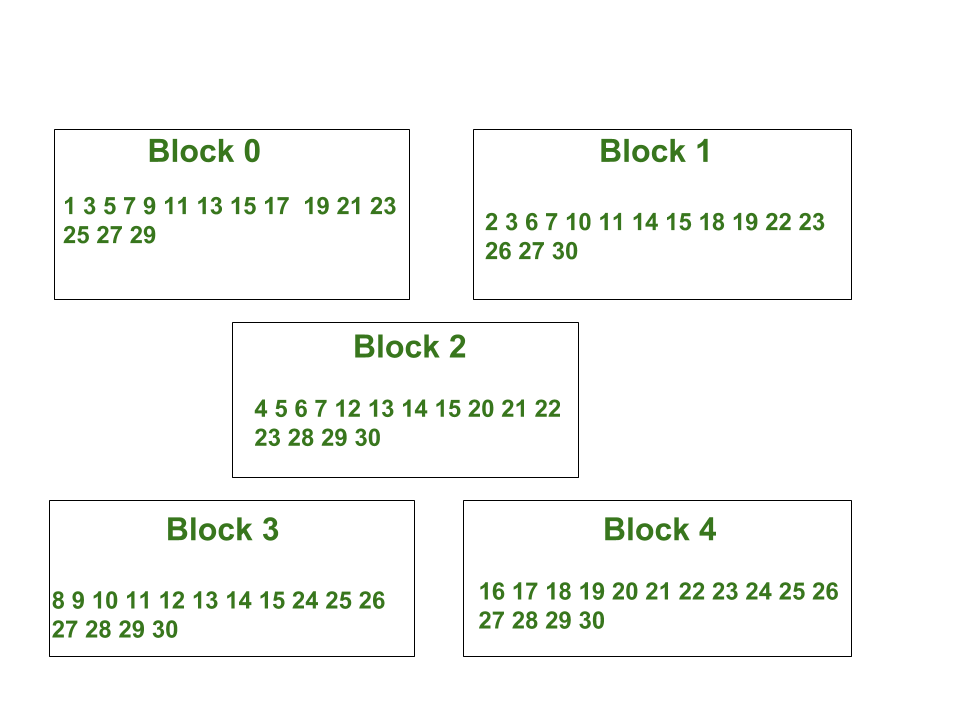# Powers of 2 to required sum

• Difficulty Level : Medium
• Last Updated : 26 May, 2022

Given an integer N, task is to find the numbers which when raised to the power of 2 and added finally, gives the integer N.

Example :

```Input : 71307
Output : 0, 1, 3, 7, 9, 10, 12, 16
Explanation :
71307 = 2^0 + 2^1 + 2^3 + 2^7 +
2^9 + 2^10 + 2^12 + 2^16

Input : 1213
Output : 0, 2, 3, 4, 5, 7, 10
Explanation :
1213 = 2^0 + 2^2 + 2^3 + 2^4 +
2^5 + 2^7 + 2^10```

Approach :
Every number can be described in powers of 2.
Example : 29 = 2^0 + 2^2 + 2^3 + 2^4.
2^0 ( exponent of 2 is ‘0’) 0
2^2 ( exponent of 2 is ‘2’) 1
2^3 ( exponent of 2 is ‘3’) 3
2^4 ( exponent of 2 is ‘4’) 4
Convert each number into its binary equivalent by pushing remainder of given number, when divided by 2 till it is greater than 0, to vector. Now, Iterate through its binary equivalent and whenever there is set bit, just print the i-th value(iteration number).

Application :
Hamming Code : Hamming Code is an error correcting code which can detect and correct one bit error. This pattern is also used in Hamming code error detection where parity bits store the XOR of numbers on the basis of LSB(Least Significant bit), where numbers are assigned in blocks and you need to find the blocks where the sum of power of 2 resulting to given number exists.

Below is the image to show the blocks with given numbers.Below is the implementation of above approach :

## C++

 `// CPP program to find the``// blocks for given number.``#include ``using` `namespace` `std;` `void` `block(``long` `int` `x)``{``    ``vector<``long` `int``> v;``    ` `    ``// Converting the decimal number``    ``// into its binary equivalent.``    ``cout << ``"Blocks for "` `<< x << ``" : "``;``    ``while` `(x > 0)``    ``{``        ``v.push_back(x % 2);``        ``x = x / 2;``    ``}` `    ``// Displaying the output when``    ``// the bit is '1' in binary``    ``// equivalent of number.``    ``for` `(``int` `i = 0; i < v.size(); i++)``    ``{``        ``if` `(v[i] == 1)``        ``{``            ``cout << i;``            ``if` `(i != v.size() - 1)``                ``cout << ``", "``;``        ``}``    ``}``    ``cout << endl;``}` `// Driver Function``int` `main()``{``    ``block(71307);``    ``block(1213);``    ``block(29);``    ``block(100);``    ``return` `0;``}`

## Java

 `// Java program to find the``// blocks for given number.``import` `java.util.*;` `class` `GFG {` `static` `void` `block(``long` `x)``{``    ``ArrayList v = ``new` `ArrayList();``    ` `    ``// Convert decimal number to``    ``// its binary equivalent``    ``System.out.print(``"Blocks for "``+x+``" : "``);``    ``while` `(x > ``0``)``    ``{``        ``v.add((``int``)x % ``2``);``        ``x = x / ``2``;``    ``}` `    ``// Displaying the output when``    ``// the bit is '1' in binary``    ``// equivalent of number.``    ``for` `(``int` `i = ``0``; i < v.size(); i++)``    ``{``        ``if` `(v.get(i) == ``1``)``        ``{``        ``System.out.print(i);``            ``if` `(i != v.size() - ``1``)``            ``System.out.print( ``", "``);``        ``}``    ``}``System.out.println();``}` `// Driver Code``public` `static` `void` `main(String args[])``{``    ``block(``71307``);``    ``block(``1213``);``    ``block(``29``);``    ``block(``100``);``}``}` `// This code is contributed by Arnab Kundu.`

## Python3

 `# Python3 program to find the``# blocks for given number.``def` `block(x):``    ` `    ``v ``=` `[]``    ` `    ``# Converting the decimal number``    ``# into its binary equivalent.``    ``print` `(``"Blocks for %d : "` `%``x, end``=``"")``    ``while` `(x > ``0``):``        ``v.append(``int``(x ``%` `2``))``        ``x ``=` `int``(x ``/` `2``)` `    ``# Displaying the output when``    ``# the bit is '1' in binary``    ``# equivalent of number.``    ``for` `i ``in` `range``(``0``, ``len``(v)):``        ``if` `(v[i] ``=``=` `1``):``            ``print` `(i, end ``=` `"")``            ``if` `(i !``=` `len``(v) ``-` `1``):``                ``print` `(``", "``, end ``=` `"")``    ``print` `(``"\n"``)` `block(``71307``)``block(``1213``)``block(``29``)``block(``100``)` `# This code is contributed by Manish``# Shaw (manishshaw1)`

## C#

 `// C# program to find the``// blocks for given number.``using` `System;``using` `System.Collections.Generic;` `class` `GFG {` `    ``static` `void` `block(``long` `x)``    ``{``        ``List<``int``> v = ``new` `List<``int``>();``    ` `        ``// Convert decimal number to``        ``// its binary equivalent``        ``Console.Write(``"Blocks for "` `+ x + ``" : "``);``        ` `        ``while` `(x > 0)``        ``{``            ``v.Add((``int``)x % 2);``            ``x = x / 2;``        ``}``    ` `        ``// Displaying the output when``        ``// the bit is '1' in binary``        ``// equivalent of number.``        ``for` `(``int` `i = 0; i < v.Count; i++)``        ``{``            ``if` `(v[i] == 1)``            ``{``                ``Console.Write(i);``                ` `                ``if` `(i != v.Count - 1)``                    ``Console.Write(``", "``);``            ``}``        ``}``    ` `        ``Console.WriteLine();``    ``}``    ` `    ``// Driver Code here``    ``public` `static` `void` `Main()``    ``{``        ``block(71307);``        ``block(1213);``        ``block(29);``        ``block(100);``    ``}``}` `// This code is contributed by Ajit.`

## PHP

 ` 0)``    ``{``        ``array_push``(``\$v``,``intval``(``\$x` `% 2));``        ``\$x` `= ``intval``(``\$x` `/ 2);``    ``}` `    ``// Displaying the output when``    ``// the bit is '1' in binary``    ``// equivalent of number.``    ``for` `(``\$i` `= 0; ``\$i` `< sizeof(``\$v``); ``\$i``++)``    ``{``        ``if` `(``\$v``[``\$i``] == 1)``        ``{``            ``print` `\$i``;``            ` `            ``if` `(``\$i` `!= sizeof(``\$v``) - 1)``                ``echo` `', '``;``        ``}``    ``}` `    ``echo` `"\n"``;``}` `// Driver Code``block(71307);``block(1213);``block(29);``block(100);` `// This code is contributed``// by Manish Shaw (manishshaw1)``?>`

## Javascript

 ``

Output:

```Blocks for 71307 : 0, 1, 3, 7, 9, 10, 12, 16
Blocks for 1213 : 0, 2, 3, 4, 5, 7, 10
Blocks for 29 : 0, 2, 3, 4
Blocks for 100 : 2, 5, 6```

Time Complexity: O(log2n)

Auxiliary Space: O(log2n)

My Personal Notes arrow_drop_up